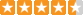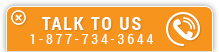# Fluid Flow Fundamentals

Course Number: M-4029
Credit: 4 PDH
Subject Matter Expert: Edward P. Brunet, Jr., P.E.
Price: \$119.80 Purchase using Reward Tokens. Details63 reviews
Overview

#### In Fluid Flow Fundamentals, you'll learn ...

• How to calculate the mass flow rate and volumetric rate for a fluid system
• The characteristics and flow velocity profiles of laminar and turbulent flow
• The relationship between the Reynolds number and the degree of turbulence of the flow
• How to use simplified and extended Bernoulli equations to solve fluid flow problems

#### OverviewPreview a portion of this course before purchasing it.

Credit: 4 PDH

Length: 56 pages

This course covers the basics of fluid flow. It begins with a basic principle of fluid flow, the conservation of mass, which leads to the continuity equation-an equation that expresses the relationship between mass flow rates at different points in a fluid system under steady-state flow conditions. The student will learn the difference between laminar and turbulent flow, how to calculate Reynolds Number, and how to do simple piping friction loss calculations.

You will also learn the difference between pressure head, velocity head, and elevation head, which are the components that comprise the Bernoulli Equation-the basic equation of fluid flow. Additionally, this course explains the differences between single-phase and two-phase flow, and it shows how a fluid system containing a centrifugal pump operates.

This course is appropriate for civil and mechanical engineers looking for a refresher course on fluid flow, as well as electrical, control systems, and structural engineers looking to expand their knowledge base.

#### Specific Knowledge or Skill Obtained

This course teaches the following specific knowledge and skills:

• Properties of fluids, including temperature, pressure, mass, specific volume, and density
• The relationship between depth and pressure
• Pascal's law concerning the effect of an external force or pressure on a confined fluid
• Volumetric flow rate and mass flow rate
• Compressibility, conservation of mass, and the continuity equation
• Differences between laminar and turbulent flow
• Calculation of Reynolds Number and friction factor
• How to use the simplified and extended Bernoulli equations
• Friction factor, head loss, and Darcy's equation
• The driving force by natural circulation in a fluid
• The relationship between flow rate and temperature difference in natural circulation flow
• Two-phase fluid flow
• How to calculate water hammer
• Operating characteristics of a centrifugal pump
• Net positive suction head (NPSH) and cavitation
• Pump laws
• Operation of centrifugal pumps in either parallel or series configuration

#### Certificate of Completion

You will be able to immediately print a certificate of completion after passing a multiple-choice quiz consisting of 30 questions. PDH credits are not awarded until the course is completed and quiz is passed.

Board Acceptance
 This course is applicable to professional engineers in: Alabama (P.E.) Alaska (P.E.) Arkansas (P.E.) Delaware (P.E.) Florida (P.E. Area of Practice) Georgia (P.E.) Idaho (P.E.) Illinois (P.E.) Illinois (S.E.) Indiana (P.E.) Iowa (P.E.) Kansas (P.E.) Kentucky (P.E.) Louisiana (P.E.) Maine (P.E.) Maryland (P.E.) Michigan (P.E.) Minnesota (P.E.) Mississippi (P.E.) Missouri (P.E.) Montana (P.E.) Nebraska (P.E.) Nevada (P.E.) New Hampshire (P.E.) New Jersey (P.E.) New Mexico (P.E.) New York (P.E.) North Carolina (P.E.) North Dakota (P.E.) Ohio (P.E. Self-Paced) Oklahoma (P.E.) Oregon (P.E.) Pennsylvania (P.E.) South Carolina (P.E.) South Dakota (P.E.) Tennessee (P.E.) Texas (P.E.) Utah (P.E.) Vermont (P.E.) Virginia (P.E.) West Virginia (P.E.) Wisconsin (P.E.) Wyoming (P.E.)
Reviews (63)
More DetailsPreview a portion of this course before purchasing it.

Credit: 4 PDH

Length: 56 pages